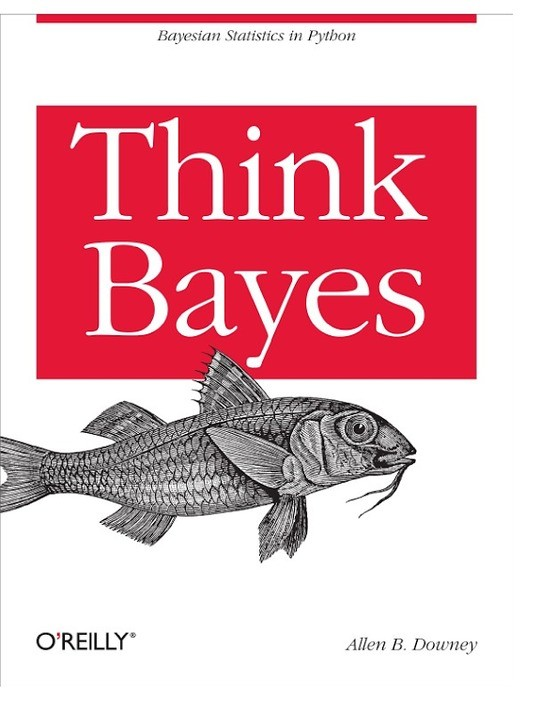Free

# Think Bayes: Bayesian Statistics Made Simple

By Allen B. Downey
Free
Book Description

Think Bayes is an introduction to Bayesian statistics using computational methods.

The premise of this book, and the other books in the Think X series, is that if you know how to program, you can use that skill to learn other topics.

Most books on Bayesian statistics use mathematical notation and present ideas in terms of mathematical concepts like calculus. This book uses Python code instead of math, and discrete approximations instead of continuous mathematics. As a result, what would be an integral in a math book becomes a summation, and most operations on probability distributions are simple loops.

The author thinks this presentation is easier to understand, at least for people with programming skills. It is also more general, because when we make modeling decisions, we can choose the most appropriate model without worrying too much about whether the model lends itself to conventional analysis. Also, it provides a smooth development path from simple examples to real-world problems.

Order print editions of Think Bayes from Green Tea Press

• Preface
• My theory, which is mine
• Modeling and approximation
• Working with the code
• Code style
• Prerequisites
• Bayes's Theorem
• Conditional probability
• Conjoint probability
• Bayes's theorem
• The diachronic interpretation
• The M&M problem
• The Monty Hall problem
• Discussion
• Computational Statistics
• Distributions
• The Bayesian framework
• The Monty Hall problem
• Encapsulating the framework
• The M&M problem
• Discussion
• Exercises
• Estimation
• The dice problem
• The locomotive problem
• An alternative prior
• Credible intervals
• Cumulative distribution functions
• The German tank problem
• Discussion
• Exercises
• More Estimation
• The Euro problem
• Summarizing the posterior
• Swamping the priors
• Optimization
• The beta distribution
• Discussion
• Exercises
• Odds
• The odds form of Bayes's theorem
• Oliver's blood
• Maxima
• Mixtures
• Discussion
• Decision Analysis
• The Price is Right problem
• The prior
• Probability density functions
• Representing PDFs
• Modeling the contestants
• Likelihood
• Update
• Optimal bidding
• Discussion
• Prediction
• The Boston Bruins problem
• Poisson processes
• The posteriors
• The distribution of goals
• The probability of winning
• Sudden death
• Discussion
• Exercises
• Observer Bias
• The Red Line problem
• The model
• Wait times
• Predicting wait times
• Estimating the arrival rate
• Incorporating uncertainty
• Decision analysis
• Discussion
• Exercises
• Two Dimensions
• Paintball
• The suite
• Trigonometry
• Likelihood
• Joint distributions
• Conditional distributions
• Credible intervals
• Discussion
• Exercises
• Approximate Bayesian Computation
• The Variability Hypothesis
• Mean and standard deviation
• Update
• The posterior distribution of CV
• Underflow
• Log-likelihood
• A little optimization
• ABC
• Robust estimation
• Who is more variable?
• Discussion
• Exercises
• Hypothesis Testing
• Back to the Euro problem
• Making a fair comparison
• The triangle prior
• Discussion
• Exercises
• Evidence
• Interpreting SAT scores
• The scale
• The prior
• Posterior
• A better model
• Calibration
• Posterior distribution of efficacy
• Predictive distribution
• Discussion
• Simulation
• The Kidney Tumor problem
• A simple model
• A more general model
• Implementation
• Caching the joint distribution
• Conditional distributions
• Serial Correlation
• Discussion
• A Hierarchical Model
• The Geiger counter problem
• Start simple
• Make it hierarchical
• A little optimization
• Extracting the posteriors
• Discussion
• Exercises
• Dealing with Dimensions
• Belly button bacteria
• Lions and tigers and bears
• The hierarchical version
• Random sampling
• Optimization
• Collapsing the hierarchy
• One more problem
• We're not done yet
• The belly button data
• Predictive distributions
• Joint posterior
• Coverage
• Discussion
The book hasn't received reviews yet.
You May Also Like
Also Available On
Categories
Curated Lists
• #### Free Machine Learning Books

11 Books

Pattern Recognition and Machine Learning (Information Science and Statistics)
by Christopher M. Bishop
Data mining
by I. H. Witten
The Elements of Statistical Learning: Data Mining, Inference, and Prediction
by Various
See more...
• #### Free Chemistry Textbooks

8 Books

CK-12 Chemistry
by Various
by Free High School Science Texts Project
General Chemistry II
by John Hutchinson
See more...
• #### Free Mathematics Textbooks

21 Books

Microsoft Word - How to Use Advanced Algebra II.doc
by Jonathan Emmons
Advanced Algebra II: Activities and Homework
by Kenny Felder
de2de
by
See more...
• #### Free Children Books

38 Books

The Sun Who Lost His Way
by
Tania is a Detective
by Kanika G
Firenze_s-Light
by
See more...
• #### Free Java Books

10 Books

Java 3D Programming
by Daniel Selman
The Java EE 6 Tutorial
by Oracle Corporation
JavaKid811
by
See more...
• #### Sts Peter And Paul Preparatory School eBook List

8 Books

Jamaica Primary Social Studies 2nd Edition Student's Book 4
by Eulie Mantock, Trineta Fendall, Clare Eastland